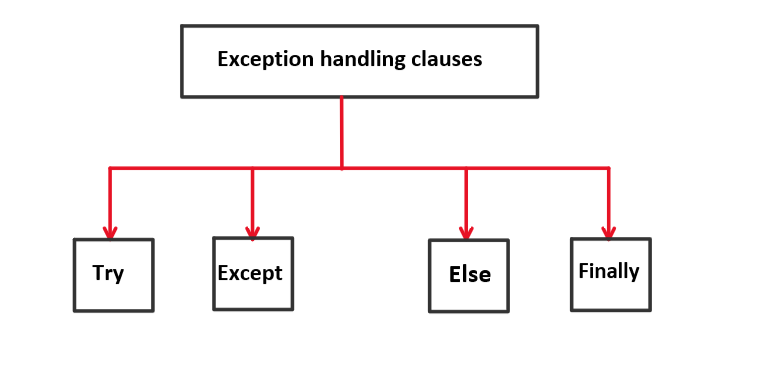# Python Exception Handling

### Introduction

If exceptions remain unhandled, they can cause abnormal termination of the program and hence confuse the programmer. In this article, we’ll learn about handling exceptions.

Generally, there are 4 clauses that we’ll use to handle the exceptions raised.### Catching and Handling exceptions – try and except statement

We can use try and except to catch and handle exceptions in python. Generally, lines of code that may produce an exception are placed within the try block and the code which will be executed when the exception occurs is placed within except block.

For example,

Code:

## Python Code

``````try:
a=12/0;
print(a);
except:
print("Division by 0 is not possible");
``````

Output:

Division by 0 is not possible

### Handling specific exceptions

We can also define the type of exception to be handled by the except statement. If we don’t specify any exception, it would handle every type of exception that would occur in the try block and would execute the print statement within it which is not a good practice

Hence from the above example, the proper code would look as follows:

```try:
a=12/0;
print(a);
except ZeroDivisionError:
print("Division by 0 not possible");```

A try statement can have any number of except statements to handle different exceptions but only one would be executed when an exception would occur. For example,

Code:

## Python Code

``````a=[1,2,3,4,5];
try:
print(a/0);
except ZeroDivisionError:
print("Division by 0 is not possible");
except IndexError:
print("Index out of bounds");
``````

Output: Division by 0 is not possible

In the above example, we created an array and tried dividing the first element with 0, hence the ZeroDivisionError exception was raised and handled by printing a message Division by 0 not possible in the shell. In the same code if we try to access the element present at the index which is not in the range of i.e. a, then the IndexError exception would be raised and handled by printing a message Index out of bounds in the shell.

else clause

What if an exception doesn’t occur, then one would want to run some other lines of code. To do this, python has a built-in clause known as the else clause. For example,

Code:

## Python Code

``````n = int(input("Enter a number: "));
try:
assert n % 2 == 0;
except:
print("Odd Number");
else :
print("Even Number");
``````

Output:

Enter a number: 2

Even Number

Enter a number: 1

Odd Number

In the above code, we are checking for even and odd numbers. If we find the given number is odd, then the assert statement would produce an exception and hence except clause would handle it and print Odd Number otherwise it would come under the else clause and would print Even Number.

### Finally clause

Very similar to the final block in java, code under the final block is executed even if the exception occurs or doesn’t occur. It is like the final point of exception handling. It is generally used to execute instructions which is a necessity.

For example, if I open a file and perform operations on that later I would close it to save resources. The closing of the file can be written under the finally clause and it would be executed at the end.

Let’s take a simpler example to understand this

Code:

## Python Code

``````n = int(input("Enter a number"));
try:
10 / n;
except ZeroDivisionError:
print("Exception occurred");
else :
print("No exception occurred");
finally:
print("Finally block is executed")
``````

Output:

Enter a number: 0
Exception occurred
Finally block is executed

Enter a number: 2
No exception occurred
Finally block is executed

In the above example if you notice, no matter what the situation is, the code under the finally clause is always executed. Hence the finally clause is super handy when you want to execute some necessary functions.